Subsections

# 7. celldetect Parameters & Data Products Reference

This chapter lists all the celldetect parameters in alphabetical order for quick reference. In addition, a guide to the data products output by celldetect is provided.

# 7.1 Default Parameter File

unix% plist celldetect

Parameters for /soft/ciao/param/celldetect.par

#
#   celldetect parameter file
#
#
#   input
#
infile =                  Input file
#
#   output
#
outfile =                  Output source list
(expstk = )                list of exposure map files
(regfile = none)            ASCII regions file
#
#   output options
#
(kernel = default)         Output file format
(clobber = no)              Overwrite exiting outputs?
#
#   output content/format options
#
(thresh = 3)               Source threshold
(findpeaks = yes)             Find local peaks?
(centroid = yes)             Compute source centroids?
(ellsigma = 3)               Size of output source ellipses (in sigmas)
(expratio = 0)               cutoff ratio for source cell exposure variation
#
#   detect cell size parameters
#
(fixedcell = 0)               Fixed cell size to use (0 for variable cell)
(xoffset = INDEF)           Offset of x axis from data center
(yoffset = INDEF)           Offset of y axis from data center
(eband = 1.4967)          Energy band
(eenergy = 0.8)             Encircled energy of PSF
(psftable = \${ASCDS_CALIB}/psfsize20010416.fits -> /soft/ciao/data/psfsize20010416.fits) Table of PSF size data
(cellfile = )                Output cell size image stack name
#
#   background parameters
#
(bkgfile = )                Background file name
(bkgvalue = 0)               Background count/pixel
(bkgerrvalue = 0)               Background error
#
#   using defaults is recommended here
#
(convolve = no)              Use convolution?
(snrfile = )                SNR output file name (for convolution only)
#
#   run log verbosity and content
#
(verbose = 0)               Log verbosity level
(log = no)              Make a celldetect.log file?
#
#   mode
#
(mode = ql)


# 7.2 Parameter Descriptions

In the following parameter descriptions, "required=yes" indicates that the user must provide a value for the indicated parameter (e.g. infile) before the program will run. Also, the empty string (" ") is equivalent to the string none for filenames.

• bkgerrvalue
Background error
type=real
def=0

The error in a background map or value.

The error in the supplied background map (bkgfile ) may be given using the bkgerrvalue parameter; if unknown, this parameter should be left at the default value (0).

• bkgfile
Background file name
type=string
filetype=input

The name of a predetermined background image for the dataset.

Instead of using celldetect 's default local detect procedure, the user may specify the background as an input image file using the parameter bkgfile . Note that if a reliable background map is available, then the user will be able to better detect extended sources which are devoid of significant brightness gradients.

This parameter is ignored if bkgvalue is nonzero.

• bkgvalue
Background count/pixel
type=real
def=0

A fixed value (events/pixels) to use for the background.

The user may instead specify the background as a fixed value of counts/pixel, using the bkgvalue parameter. Be aware that if this value is too low, spurious sources will be detected on the periphery of the dataset.

A non-zero value for bkgvalue overrides the normal background estimation and the background file (bkgfile ) option.

• cellfile
Output cell size image stack name
type=string
filetype=output
autoname=yes

The name of a stack for file names of cell size images.

If a filename is provided for the cellfile parameter, then one or more images are created which contain the detect cell size at each pixel location. If autonaming is used (i.e. "cellfile=."), then the output stack file will be named "<infile_root>_cell".

• centroid
Compute source centroids?
type=boolean
def=yes

Compute source centroid positions?

If centroid is set to yes (the default), then the source list will be based on the source centroid. In addition, the major and minor axes of the events distribution are calculated as well as the position angle of the major axis. However, if centroid is set to no, then the center pixel of a source's detect cell is reported as the location of the source.

• clobber
Overwrite exiting outputs?
type=boolean
def=no

If "yes", overwrite existing outputs.

celldetect does not not overwrite existing outputs unless clobber is set to yes.

• convolve
Use convolution?
type=boolean
def=no

If "yes", detection is done using the convolution library instead of sliding cells.

Setting convolve to yes will cause detection to be done using the convolution library instead of sliding cells (the default). This method is much slower, does not work with recursive blocking, and should really only be used when an output S/N map is needed.

The convolve option produces a pixel-by-pixel map of the S/N, which is written to the file specified by snrfile .

• eband
Energy band
type=real
def=1.4967
units=keV

The photon energy (keV) at which the point spread function (PSF) is chosen.

The selection of a particular PSF size is governed by the energy band eband parameter [keV], since the Chandra PSF is a function of energy. By default the energy band is set to 1.4967 keV.

• eenergy
Encircled energy of PSF
type=real
def=0.8
max=1.0

The percentage of PSF energy to be encircled by the detect cell, expressed as a fraction of 1.0.

celldetect also uses an encircled energy percentage value, given by the eenergy parameter, in concert with the calibration PSF file. This value is the percentage of PSF energy to be encircled by the detect cell, expressed as a fraction of 1.0 (default is 0.8). The eenergy is a key parameter for detecting off-axis sources, and users are urged to experiment with other values.

• ellsigma
Size of output source ellipses (in sigmas)
type=real
def=3.0

The size, in sigmas, to make the elliptical source detection regions.

The size of the elliptical source regions in both of the source region files (regfile and outfile ) is controlled via the ellsigma parameter. This parameter is a multiplicative factor applied to sigma, the standard deviation of the distribution, to scale the major and minor axes of the ellipses for each source detection.

The distribution is defined as the distribution of counts within the source cell (i.e. the observed counts), and is assumed to be Gaussian. Thus, if ellsigma is 1, then the elliptical source region will contain 39.3% of the source counts (i.e. the 1-sigma point for a 2-D Gaussian).

This feature is included so that the graphics overlay may be made more visible; it is does not affect the detections themselves, only the display of the regions.

• expratio
Cutoff ratio for source cell exposure variation
type=real
def=0

Suppresses ASCII source regions for sources whose EXPO_RATIO falls below this value.

The expratio parameter is the ratio of the average exposure of the background frame to the average exposure in the detect cell. Exposure maps supplied by the user are used in conjunction with the expratio parameter. For expratio values other than 0, exposure maps must be supplied in the expstk parameter. Detections for sources whose ratio falls below the specified expratio value will be suppressed from the source region file (regfile ). However, all detections will be present in the output source list (outfile ), and the column EXPO_RATIO will be created. Recommended expratio values ares between 0.9 and 0.99.

• expstk
List of exposure map files
type=string
filetype=input
stacks=yes

The name of an exposure map stack or file.

To minimize spurious detections along detector edges, one or more exposure maps may be supplied. The expstk parameter takes a stack of exposure map filenames; sse "ahelp stack'' for more information. Each of the exposure map files should have appropriate binning to match celldetect 's recursive blocking (i.e. binning by 2, 4, 8, ...). Exposure maps only work with the local detect procedure. In addition, they do not work with the convolution option.

• findpeaks
Find local peaks?
type=boolean
def=yes

Find the local maxima of contiguous detections?

If the findpeaks parameter is set to no, then the source list will consist of all detection cells which exceeded the S/N threshold (often resulting in single source being identified multiple times). If findpeaks is set to yes (the default), then adjacent detections are recognized as a single source and the cell with the largest S/N appears in the source list.

• fixedcell
Fixed cell size to use (0 for variable cell)
type=integer
def=0
units=pixels

A fixed cell size, which celldetect will use over the complete field of view.

celldetect has a fixed cell size option, where the detect cell size is kept constant for the entire dataset. Choose the cell size in pixels by setting the fixedcell parameter; allowed values are 1 or an integer divisible by 3. Setting fixedcell to a non-zero value effectively disables the parameters related to celldetect 's variable cell size functionality: psftable , eenergy , eband , xoffset , and yoffset parameters.

If celldetect is to be run on non-Chandra data, supply a value for fixedcell .

Fixed cell size is also useful for extended source detection. It is well known that local detect methods are not very useful for significantly extended sources. To overcome this limitation, the user may either set fixedcell to a size sufficient to match the expected size of an extended source, or employ a background map (or fixed value for the background) instead of using local detect.

• infile
Input file
type=string
filetype=input
required=yes

The name of the input file.

The input FITS file can an event list or an image. CIAO Data Manipulation (DM) syntax may be used in the input file specification, as explained in Chapter 1, Section 1.3.3. For a FITS event list, celldetect will automatically find the EVENTS extension; otherwise the extension should be specified using DM syntax (e.g. "data.fits[EVENTS]").

The current maximum size allowed for an image is 2048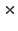2048, but an event list may occupy a larger spatial region. For event list datasets larger than 20482048 (as given by TLMIN, TLMAX), celldetect may use the "recursive blocking scheme": First the inner 20482048 pixel region of the dataset is searched for sources, then the inner 40964096 pixel region (excluding the area already analyzed) is blocked by 2 and searched for sources, then the inner 81928192 is blocked by 4, etc. , until TLMIN, TLMAX is reached. For each consecutive search, the portion of the data that has already been searched for sources in higher resolution is skipped, i.e. only one blocking factor is used for each region of the dataset.

• kernel
Output file format
type=string
def=default

The format for the output file.

The kernel parameter controls the output format (default, fits, or iraf); the default is for the output file to have the same format as the input file.

• log
Make a celldetect.log file?
type=boolean
def=no

The verbosity of log information is sent to STDERR (usually the screen). However, if log is set to yes, then the log information will be written to the file celldetect.log.

• mode
def=ql

The mode defines how to handle querying for parameters. For example, setting the mode to "h'' means that no prompting will occur; this is useful when running the tool from a script. See "ahelp parameter'' for more information and a list of all the available modes.

• outfile
Output source list
type=string
filetype=output
required=yes
autoname=yes

The name of the output file.

The outfile parameter is used to provide a name for the output file. The kernel parameter controls the output format; the default is for the output file to have the same format as the input file. If autonaming is used (i.e. "outfile=."), then the output file will be named "<infile_root>_src".

• psftable
Table of PSF size data
type=string
filetype=ARD
def=psfsize20010416.fits

The path and name of the file containing PSF information.

celldetect compensates for the larger Chandra PSF off-axis by using a "variable cell size" technique, which increases the size of the detect cell with off-axis distance. The PSF information used by celldetect is contained in a Chandra calibration file distributed with CIAO ; the location is given by the value of the psftable parameter, which points by default to the appropriate Chandra calibration PSF file.

The Chandra HRC and ACIS detectors are the sole combinations in the PSF library file used by celldetect ; information for other missions is not provided. celldetect can only utilize the variable cell size procedure if the following keywords are present in the input Chandra data file (infile ): TELESCOP, INSTRUMENT, DETNAM, GRATING, RA_NOM, and DEC_NOM. If any of the keywords are missing, the tool cannot calculate the appropriate cell size and fixedcell needs to be specified instead.

• regfile
ASCII region file
type=string
autoname=yes

The name of an ASCII source region output file.

In addition to the output source list (outfile ), the user may choose to have an ASCII source region file written by specifying a filename in regfile parameter. If autonaming is used (i.e. "regfile=."), then the source regions output file will be named "<infile_root>_reg".

This region file contains the location and size of the principal axes, for each detected source elliptical region. The elliptical region size is such that the region contains some fraction of the source counts. The parameter ellsigma specifies the desired source count fraction.

If an exposure map has been used, and expratio is non-zero, there may be fewer entries in regfile than in outfile .

This region file is useful for subsequent input into ds9, particularly to overlay region markers over each detection; see Chapter 1, Section 1.4 for details.

• snrfile
SNR output file name (for convolution only)
type=string
filetype=output
autoname=yes

The name of a signal-to-noise (S/N) map output file.

The convolve option produces a pixel-by-pixel map of the S/N, which is written to the file specified by snrfile . A S/N value is computed for every pixel in the input dataset, not just for source locations. The file snrfile is then an image of these values. If autonaming is used (i.e. "snrfile=."), then the S/N output file will be named "<infile_root>_snr".

• thresh
Source threshold
type=real
def=3

The signal-to-noise (S/N) threshold for source detection.

The detection threshold, thresh , is the signal-to-noise (S/N) cutoff for source detection, and should be set to a value of 3 (the default) or 4, particularly for the initial run.

• verbose
Log verbosity level
type=integer
def=2
min=0
max=5

The verbosity of log output.

The verbosity of log information ranges from 0 (none) to 5 (maximum output) and is sent to STDERR (usually the screen).

• xoffset
Offset of x axis from data center
type=integer
def=INDEF
units=pixels

The offsets of the x-axis for the calculation of the off-axis angle.

By default, celldetect calculates the off-axis angle using the nominal pointing of the data file as the origin. Users may change this behavior by setting offsets to any numerical values (using the xoffset and yoffset parameters); these offsets provide the location of the optical axis with respect to the center of the data file. A typical scenario in which the user may select to use offsets is when the data file is a sum of two or more observations with different pointings. The nominal pointing in such a data file is usually poorly defined and the off-axis angle could be calculated from an undesired origin, leading to suboptimal selection of the detect cell size.

• yoffset
Offset of y axis from data center
type=integer
def=INDEF
units=pixels

The offsets of the y-axis for the calculation of the off-axis angle.

See the description for xoffset .

# 7.3 Data Product Descriptions

Source List File (outfile )

The primary output of celldetect is the FITS file of source detections. If the signal-to-noise (S/N) ratio in a detect cell exceeds the threshold set by the user (thresh ), then the cell location is considered a source candidate and the following properties are recorded in the output file: 7.1

 Data item Unit Description RA deg Source right ascension DEC deg Source declination RA_ERR deg Source right ascension error DEC_ERR deg Source declination error POS(X,Y) pixel Physical coordinates X_ERR pixel Source X position error Y_ERR pixel Source Y position error CELLPOS(CELL_X,CELL_Y) pixel Physical coordinates DETSIZE pixel Source cell size BKGSIZE pixel Background cell size NPIXSOU pixel Pixels in source cell NPIXBKG pixel Pixels in background cell NET_COUNTS count Net source counts NET_COUNTS_ERR count Error in net source counts BKG_COUNTS count Background counts (scaled to source cell) BKG_COUNTS_ERR count Error in background counts NET_RATE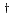count/s Source count rate NET_RATE_ERRcount/s Source count rate error BKG_RATEcount/s Background count rate BKG_RATE_ERRcount/s/pixel Background count rate error EXPTIMEs Effective exposure time SNR Signal-to-noise ratio SHAPE Shape of source region R Radii of source region ROTANG deg Rotation angle of source region PSFRATIO Ratio of source ellipse size to PSF size BLOCK Blocking factor COMPONENT Source number EXPO_RATIO Ratio of average background and detect cell exp DOUBLE Double detection flag DOUBLE_ID Double source component

• RA, DEC:
Right ascension and declination coordinates, corresponding to X and Y. Calculated only if WCS keywords are present in the data, otherwise filled with zeros.

• RA_ERR, DEC_ERR:
RA and DEC errors, corresponding to X_ERR and Y_ERR. Calculated only if input parameter centroid is set to yes, otherwise "INDEF''.

• POS(X,Y):
Location of the detect cell. If input parameter centroid is set to yes, then X and Y are the centroid of photon distribution in the detect cell. If centroid is set to no, then X and Y are the location of the cell.

• X_ERR, Y_ERR:
Uncertainties on the centroid location. Calculated only if input parameter centroid set to yes, otherwise filled with INDEF.

• CELLPOS(CELL_X,CELL_Y):
The center position of the detect cell.

• DETSIZE:
Source cell size. The final cell size must be unity or an integer which is divisible by 3. See also NPIXSOU.

• BKGSIZE:
If either background map (bkgfile ) or background value (bkgvalue ) are provided then this value is equal to DETSIZE or NPIXSOU, respectively.

If background map and background value are not provided then the background is estimated from the input data by calculating events in the frame surrounding the detect cell. The size of the background frame is calculated from DETSIZE by multiplying by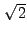and then rounding it (up or down) to the closest number of the same parity as the detect cell size. (In that way two goals are achieved: first, the area of the background frame is roughly the same as the area of the detect cell, and second, the background frame is centered on the same location as the detect frame.)

• NPIXSOU:
The actual number of image pixels contained in the source cell; square of DETSIZE.

• NPIXBKG:
The actual number of pixels in the background frame. If the background frame is entirely within the dataset, then NPIXBKG is equal to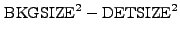; it is reduced appropriately if the background frame falls outside the dataset. See also BKGSIZE.

• NET_COUNTS:
Counts in the detect cell reduced by the number of background counts scaled to the detect cell.

• NET_COUNTS_ERR:
Estimated uncertainty of NET_COUNTS.

• BKG_COUNTS:
Background counts scaled to the detect cell area.

• BKG_COUNTS_ERR:
Estimated uncertainty of BKG_COUNTS.

• NET_RATE:
Source count rate: the NET_COUNTS divided by EXPTIME. Not implemented at present; filled with zeros.

• NET_RATE_ERR:
Source count rate corresponding error: the NET_COUNTS_ERR divided by EXPTIME. Not implemented at present; filled with zeros.

• BKG_RATE:
Background count rate: simply the BKG_COUNTS divided by EXPTIME. Not implemented at present; filled with zeros.

• BKG_RATE_ERR:
Background count rate corresponding error: simply the BKG_COUNTS_ERR divided by EXPTIME. Not implemented at present; filled with zeros.

• EXPTIME:
Effective exposure time in the detect cell, taken from the exposure map. Not implemented at present; filled with zeros.

• SNR:
The calculated signal-to-noise ratio in the detect cell.

• SHAPE:
The shape of region output to the ASCII source region file. If centroid is yes then SHAPE is ellipse; if centroid is no then SHAPE is box.

• R:
The estimated semi-major and semi-minor axis lengths (i.e. the x and y radius lengths in pixels, before rotation). For SHAPE = ellipse, this is ellsigma times the axis lengths. For SHAPE = box, this is ellsigma times the cell side lengths.

• ROTANG:
The counterclockwise angle between the semi-minor axis (see R) and the y-axis of the image, in degrees.

• PSFRATIO:
PSFRATIO is defined as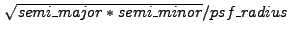where psf_radius is the radius at the source off-axis angle which corresponds to eenergy .

• BLOCK:
For event list datasets larger than 20482048, celldetect may use the "recursive blocking scheme". The value of BLOCK provides the information on the blocking factor used at the position of the source.

• COMPONENT:
The number assigned to the detected source. The source numbers correspond to those in the output source list. Its primary use is for filtering of the source list by CIAO tools (e.g. "component=1:10", "component=1,2,5").

• EXPO_RATIO:
When an exposure map is input (expstk ), celldetect computes the average exposure found in the detect cell and background frame. EXPO_RATIO is the ratio of these two average exposures.

• DOUBLE:
When DOUBLE is TRUE, this detection is believed to be a duplicate of a source detected in a different recursive blocking pass.

• DOUBLE_ID:
The component of the source for which this detection is believed to be a duplicate.

ASCII Source Region File (regfile )

In addition to the source list file (outfile ), the user may also select to have a source region file (regfile ) output.

This region file is useful for subsequent input into ds9, particularly to overlay region markers over each detection; see Chapter 1, Section 1.4 for details.

The source region file from the example in Chapter 4, Section 4.2.2:

unix% more example2_out.reg
ellipse(251.954128,268.889908,3.688666,2.832946,93.695023)
ellipse(252.031008,309.038760,3.638202,3.090788,103.071884)
ellipse(251.955556,320.983333,3.917530,3.097792,98.666176)
.
.
.


Log File (logfile )

If log is set to yes, then the log information will be written to the file celldetect.log.

The log file from the example in Chapter 4, Section 4.2.2:

unix% more celldetect.log
input file: data/datasetA.fits[123:630,109:614]
output file: example2_out.fits
ASCII source regions file: example2_out.reg
creating cell size table
creating candidate source list
cell size 3
cell size 6
cell size 9
cell size 12
Writing out cell file: datasetA_cell_1.fits


Cell Size Image (cellfile )

If a filename is provided for the cellfile parameter, then one or more images are created which contain the detect cell size at each pixel location (see Example 4.2.2). Pixels in the cell size image have values equal to the cell size used to search for sources at those pixel locations.

Signal-to-noise Ratio Map (snrfile )

The convolve option produces a pixel-by-pixel map of the S/N, which is written to the file specified by snrfile . A S/N value is computed for every pixel in the input dataset, not just for source locations. The file snrfile is then an image of these values.